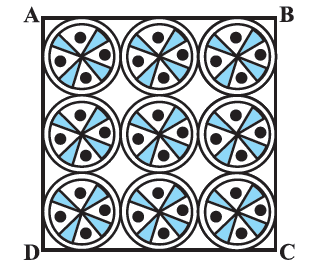# Ex.12.3 Q11 Areas Related to Circles Solution - NCERT Maths Class 10

Go back to  'Ex.12.3'

## Question

On a square handkerchief, nine circular designs each of radius $$7\, \rm{cm}$$ are made (see Figure). Find the area of the remaining portion of the handkerchief.Video Solution
Areas Related To Circles
Ex 12.3 | Question 11

## Text Solution

What is known?

On a square handkerchief, $$9$$ circular designs each of radius $${(r)} = \rm7cm$$ are made.

What is unknown?

Area of the remaining portion of the handkerchief.

Reasoning:

Radius of circular design $$\text{= 7 cm}$$

$$\therefore \;$$Diameter each circular design $$=\,7 \times 2 = 14{\text{cm}}$$

Visually and logically since all the $$3$$ circular design are touching each other and cover the entire length of the square.

$$\therefore \;$$Side of the square(s)= three times diameter of circular design

\begin{align}= 3 \times 14cm = 42cm\end{align}

Also, visually from the figure it is clear that:

Area of the remaining portion of handkerchief  $$=$$  Area of square $$- \,\,9 \times$$ (Area of each circular design)

$= {s^2} - 9\left( {\pi {r^2}} \right)$

Which can be easily solved since side $$(s)$$ of square and radius $${(r)}$$ are known.

Steps:

Radius of each circular design $$\rm{}r = 7\; \rm{cm}$$

$$\because\;$$ Diameter of each circular design $$\rm{}= 2r =2\times 7\,cm=\text{ 14 cm}$$

From the figure, it is observed that

Side of the square \begin{align}s = 3 \times 14cm = 42cm\end{align}

\begin{align}&= 14 \,\rm{cm}\, + 14 cm + 14 \,\rm{cm}\\ &= 42\,\rm{cm}\end{align}

Area of the remaining portion of the handkerchief = Area of square $$- \,9\; \times$$  (Area of each circular design)

\begin{align} &={{s^2} - 9\left( {\pi {r^2}} \right)}\\ &= {{{(42)}^2} - 9 \times \frac{{22}}{7} \times {{(7)}^2}}\\&= {1764 - 9 \times 22 \times 7}\\ &= {1764 - 1386}\\&= {378\,\,{\text{c}}{{\text{m}}^2}}\end{align}

Learn from the best math teachers and top your exams

• Live one on one classroom and doubt clearing
• Practice worksheets in and after class for conceptual clarity
• Personalized curriculum to keep up with school